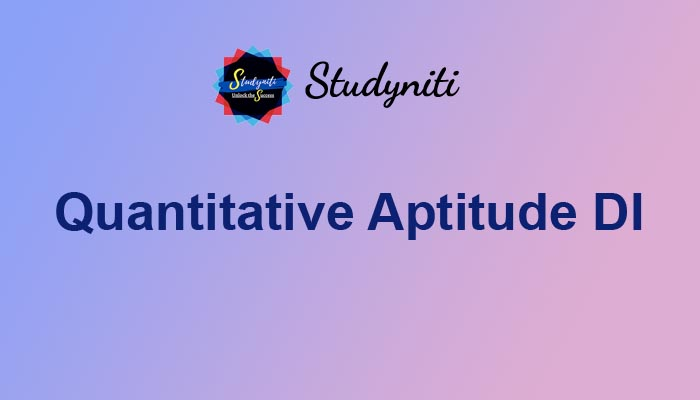## Payment Processing...# Quantitative Aptitude DI - Nov 17 2020

Here we are providing new series of Quantitative Aptitude Questions for upcoming exams, so the aspirants can practice it on a daily basis.

Study the following information carefully and answer the questions given below.

The given bar graph shows the number of students from 6 different colleges.1) Ratio of the number of boys to girls from A is 5: 4. If the hostel fee for a girl is Rs.10000 and a boy is Rs.12000, then find the total hostel fee of both boys and girls?

A.30 lakh

B.20 lakh

C.40 lakh

D.60 lakh

E.None of these

2) What is the average number of students from B, C, D, E and F together?

A.400

B.401

C.402

D.404

E.None of these

3) The ratio of the number of boys to girls from B and E is 7:8 and 5:3 respectively. The number of girls from B and E together is approximately what percent of the number of boys from B and E together?

A.82%

B.85%

C.89%

D.92%

E.None of these

4) The number of students from C is what percent of the total number of students from D?

A.40%

B.50%

C.55%

D.45%

E.None of these

5) What is the ratio of the average of the students from A, B and F together to the average number of students from C, D and E together?

A.31:41

B.16:21

C.33:43

D.35:44

E.None of these

Answers :

1) Answer: C

Boys from A = 5/9 * 360 = 200

Girls from A = 4/9 * 360 = 160

Total hostel fee of boys = 200 * 12000 = 2400000

Total hostel fee of girls = 160 * 10000 = 1600000

Total revenue = 2400000 + 1600000 = 4000000 = 40 lakh

2) Answer: C

Average = (450 + 280 + 560 + 480 + 240)/5

= 402

3) Answer: A

Number of boys from B = 450 * 7/15 = 210

Number of girls from B = 450 * 8/15 = 240

Number of boys from E = 5/8 * 480 = 300

Number of girls from E = 3/8 * 480 = 180

Required percentage = (240 + 180)/(300 + 210) * 100

= 82%

4) Answer: B

Required percentage = (280/560) * 100 = 50%

5) Answer: D

Required ratio = (360 + 450 + 240)/3:(280 + 560 + 480)/3

=350: 440

= 35: 44